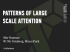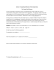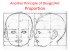# STAT 100, Section 4 Sample Final Exam Questions Fall 2008

## Transcription

STAT 100, Section 4 Sample Final Exam Questions Fall 2008
```STAT 100, Section 4
Sample Final Exam Questions
Fall 2008
The following questions are similar to the types of questions you will see on the final
exam. The actual final will consist of 70 to 80 multiple-choice questions.
NOTE: On the actual exam, the choices will not require calculator math.
On this sample exam, some of the choices may require a calculator.
Chapter 1
Question 1. The importance of randomized experiments is that they:
(A) Require only small sample sizes.
(B) Allow the statistician to assign units to the treatment group.
(C) Allow the inference of causation.
(D) None of the above.
Question 2. In a statistical study, the sample is:
(A) The group of people or objects for which conclusions are to be made.
(B) A subset of people in the United States.
(C) The subset of the population on which the study collects data.
(D) The collection of data in sample surveys.
Question 3. To estimate the unemployment rate, the Bureau of Labor Statistics contacts
about 60,000 households chosen randomly from a list of all known households. The
Bureau asks each adult in every sampled household whether they are in the labor
force, i.e., employed or seeking employment. The population of interest is:
(A) All known or unknown households.
(B) All adults who are in the labor force.
(D) All adults who refused to participate in the survey.
Question 4. In a statistical study, the population is:
(A) The group of people from whom data cannot be collected.
(B) The people or objects studied in the sample survey.
(C) The group of people or objects for which conclusions are to be made.
(D) All people in the United States.
Chapter 3
1
Question 5. An advertiser of No-Pain aspirin claims it is the pain-killer most preferred
by consumers. This claim was based on a consumer survey in which the choices were:
Lavid, Acinna, No-Pain, and Yellnot. This is an example of:
(A) An easy question.
(B) An open question.
(C) A difficult question.
(D) A closed question.
Question 6. When measured with extreme accuracy, the variable “height of a building”
is:
(A) A discrete quantitative variable
(B) A nominal categorical variable
(C) A continuous quantitative variable
(D) An ordinal categorical variable
Question 7. Which of the following measures is valid and categorical?
(A) Time on a clock that is always 10 minutes fast
(B) The sale price of a house
(C) Sex (male or female)
(D) Weight of an individual on a scale that is sometimes 5 pounds too light, sometimes 5
pounds too heavy
Question 8. In a study of car ownership in Pennsylvania, the variable “Brand of car
owned” is:
(A) A discrete quantitative variable
(B) A nominal categorical variable
(C) A continuous quantitative variable
(D) An ordinal categorical variable
Chapter 4
Question 9. The Gallup Poll, a well-known polling group, regularly surveys the public on many issues. The number of interviews on which their surveys are based is,
approximately:
(A) 60,000 – 120,000
(B) 60–120
(C) 600,000 – 1,200,000
(D) The entire U.S. population.
(E) 600 – 1,200
2
Question 10. To survey the opinions of its customers, a supermarket grouped its customers by the days when they did most of their shopping. The supermarket randomly
selected two such groups, and asked all customers in those two groups to complete a
survey. This (biased) method of sampling is called:
(A) Stratified random sampling.
(B) Systematic sampling.
(C) Random digit dialing.
(D) Cluster sampling.
Question 11. A radio advertiser wishes to choose a sample of size 100 from a population
of 5000 listeners. He divides the population into five separate groups and then selects
a simple random sample from each group. This method of sampling is called:
(A) Simple random sampling.
(B) Systematic random sampling.
(C) Volunteer sampling.
(D) Stratified random sampling.
Question 12. To obtain a margin of error of 1% we need a sample of size:
(A) 1,000
(B) 100,000
(C) 10,000
(D) 100
Question 13. A simple random sample of ten subjects from a population is one in which:
(A) Any group of ten subjects has the same chance of being the selected sample.
(B) A simple way was found to choose ten subjects at random from the population.
(C) Most, but not all, groups of size ten have the same chance of being selected.
(D) We record the data provided by the first ten subjects who respond to the survey.
Question 14. A radio advertiser wishes to choose a sample of size 100 from a population
of 5000 listeners. He divides the population into a large number of groups. He selects
a simple random sample of the groups and then surveys every subject in each of the
groups selected. This method of random sampling is called:
(A) Cluster sampling.
(B) Random digit dialing.
(C) Stratified random sampling.
(D) Systematic random sampling.
3
Question 15. A supermarket manager wants to know if customers would pay slightly
higher prices to have computers available throughout the store to help them locate
items. An interviewer is posted at the entrance door and asked to collect a sample of
100 opinions by asking questions of the next person who came to the door each time
she had completed an interview. This method of sampling is called:
(A) Cluster sampling.
(B) Stratified random sampling.
(C) Random digit dialing.
(D) Convenience sampling.
(E) Systematic sampling.
Question 16. A radio advertiser wishes to choose a sample of size 100 from a population
of 5000 listeners. After observing that 5, 000 ÷ 50 = 100, he first selects a subject at
random from the first 50 names in the sampling frame, and then he selects every 50th
subject listed after that one. This method of sampling is called:
(A) Stratified random sampling.
(B) Simple random sampling.
(C) Cluster random sampling.
(D) Systematic random sampling.
Question 17. In a study of PSU students’ awareness of world issues, a television crew
sampled students sitting in the Hub at 12:00 p.m. (noon). This survey is an example
of:
(A) A simple random sample, because it is simple to find students at the Hub in a random
manner.
(B) A haphazard (or convenience) sample, because the Hub is a convenient place to find
students.
(C) A stratified random sample, because students are stratified by gender and they are
found at the Hub in a random manner.
(D) A volunteer response sample because, to be seen on the evening news, students will
eagerly volunteer their responses.
Question 18. If a simple random sample of 1,600 subjects is chosen then an approximate
margin of error for making inferences about a percentage of the entire population is:
(A) 1/1, 600, or 0.06%.
(B) Impossible to calculate without knowing the percentage observed in the sample.
(C) Very high, because the sample size is a tiny percentage of the population size.
(D) Approximately zero because the sample size is so large.
√
(E) 1/ 1, 600, or 2.5%.
Chapter 5
4
Question 19. To test the effects of sleepiness on driving performance, twenty volunteers
took a simulated driving test under each of three conditions: Well-rested, Sleepy, and
Exhausted. The order in which each volunteer took the three tests was randomized,
and an evaluator rated their driving accuracy without knowing the condition of the
volunteer. This type of experiment is a:
(A) Single-blind, matched-pair experiment.
(B) Single-blind, block design experiment.
(C) Retrospective observational study.
(D) Double-blind, matched-pair experiment.
Question 20. The term “control” means that:
(A) There must be a basis for making comparisons.
(B) We control carefully the cost of performing the experiment.
(C) We control carefully the observed outcomes of the experiment.
(D) There is a need to control the subjects of the experiment.
Question 21. It has been observed that participants in a statistical experiment sometimes
respond differently than they otherwise would because they know that they are in an
experiment. This phenomenon is called the:
(A) Interacting effect.
(B) Confounding effect.
(C) Placebo effect.
(D) Hawthorne effect.
Question 22. To test the effects of sleepiness on driving performance, twenty volunteers
took a simulated driving test under each of three conditions: Well-rested, Sleepy, and
Exhausted. The order in which each volunteer took the three tests was randomized,
and an evaluator rated their driving accuracy without knowing the condition of the
volunteer. The explanatory variable is:
(A) The order in which the volunteers took the tests.
(B) The condition of sleepiness.
(C) The evaluator’s rating of the driver’s condition.
(D) The evaluator’s rating of driving accuracy.
Question 23. The term “randomization” means that we:
(A) We study only a random subset of all observed outcomes of the experiment.
(B) Allow subjects to assign themselves randomly to the placebo or treatment group.
(C) Use a chance mechanism to assign subjects to the treatment and control groups.
(D) Assign the subjects to the random experiment in a systematic manner.
5
Question 24. A researcher asks 1,600 randomly chosen doctors whether or not they take
aspirin regularly. She also asks them to estimate the number of headaches they have
had in the past six months, and compares the number of headaches reported by those
who take aspirin regularly to the number of headaches reported by those who do not
take aspirin regularly. In this study, the number of headaches is a:
(A) Response variable.
(B) Explanatory variable.
(C) Confounding variable.
(D) None of the above.
Question 25. In 1982, 490,000 subjects were asked about their drinking habits. Researchers tracked subjects’ death rates until 1991, and found that adults who regularly
had one alcoholic drink daily had a lower death rate than those who did not drink.
Most of the subjects were middle-class, married, and college-educated.
This experiment was:
(A) A randomized experiment; subjects were assigned in a randomized manner to have
one alcoholic drink each day.
(B) An observational study; it would not be ethical for the researchers to randomly assign
subjects to drink alcohol or not.
(C) Based on a stratified random sample; subjects were stratified randomly into overlapping groups according to whether or not they had one drink daily.
(D) Accurate; there is fundamentally solid anecdotal evidence that people’s health will
improve if they have one drink daily.
Question 26. In 1982, 490,000 subjects were asked about their drinking habits. Researchers tracked subjects’ death rates until 1991, and found that adults who regularly had one alcoholic drink daily had a lower death rate than those who did not
drink. Most of the subjects were middle-class, married, and college-educated. This
experiment is an example of:
(A) A block design; many groups were compared, e.g., married vs. not married, middleclass vs. not middle-class.
(B) A prospective study; after recording their drinking habits, subjects were studied into
the future.
(C) A matched pairs design; two groups were compared, those who had one drink daily
and those who did not.
(D) A retrospective study; after the nine-year period, survivors were asked about their
drinking habits.
Question 27. A researcher asks 1,600 randomly chosen doctors whether or not they take
aspirin regularly. She also asks them to estimate the number of headaches they have
had in the past six months, and compares the number of headaches reported by those
who take aspirin regularly to the number of headaches reported by those who do not
take aspirin regularly. This type of study design is a:
(A) Retrospective observational study.
(B) Randomized experiment.
(C) Census.
(D) Prospective study.
6
Question 28. It has been observed that participants in a statistical experiment sometimes
respond positively to a placebo, a substance which has no active ingredients. This
phenomenon is called the:
(A) Placebo effect.
(B) Interacting effect.
(C) Hawthorne effect.
(D) Confounding effect.
Chapter 7
Question 29. For any data set, the proportion of the data that falls at or above the
median is:
(A) 95%
(B) 99.7%
(C) 25%
(D) 50%
(E) Dependent on the sample which was drawn from the population.
Question 30. When a distribution is greatly skewed to the right (i.e., has a long right
tail but a comparitively short left tail), the median will usually be:
(A) In no relation whatsoever to the mean.
(B) Smaller than the mean.
(C) Larger than the mean.
(D) Exactly equal to the mean.
Question 31. A sample of 28 temperature measurements in ◦ F, all taken at 12:00 p.m.,
was collected in a coastal town in NC. A second sample of 28 temperature readings
in a GA coastal town was also collected. Each GA measurement was recorded at the
same time and date as the NC data value, and turned out to be exactly 5◦ F higher
than the corresponding NC measurement. We calculate the standard deviation (S.D.)
of the two data sets and conclude that:
(A) The two data sets have the same standard deviations.
(B) The S.D. of the NC data exceeds the S.D. of the GA data by 5◦ F.
(C) The S.D. of the GA data exceeds the S.D. of the NC data by 5◦ F.
(D) There is not enough information to determine the relationship between the two S.D.’s.
Question 32. For any data set, the largest number in the five-number summary is the:
(A) Third quartile.
(B) Maximum.
(C) Standard deviation.
(D) Outlier.
(E) Interquartile range.
7
Question 33. In a boxplot (oriented vertically, with the maximum at the top and the
minimum at the bottom), the proportion of the data falling between the top edge and
the bottom edge of the box itself is
(A) 25%
(B) 99.7%
(C) 50%
(E) 68%
Question 34. A sample of 28 temperature measurements in ◦ F, all taken at 12:00 p.m.,
was collected in a coastal town in NC. The data are given in the following stemplot:
3
5
6
7
8
9
2
5
0
3
0
0
1
5
0
2
2
5
2
3
4
6
3
5
48
8899
458
8
For the data given in this stemplot, the five-number summary is:
(A) 55, 66, 78.5, 84.5, 95
(B) 32, 68, 79, 88, 98
(C) 32, 66, 78.5, 84.5, 98
(D) 32, 66, 78, 84, 98
Question 35. For any data set, the standard deviation is:
(A) A measure of central tendency.
(B) The average of the deviations.
(C) A measure of the spread or variability of the data.
(D) The average of the sample mean and quartiles.
Question 36. If a particular dataset has a five-number summary given by 10, 20, 30, 40,
50, then the interquartile range is:
(A) 30 − 5 = 25
(B) 40 − 20 = 20
(C) (10 + 20 + 30 + 40 + 50)/5 = 30
(D) 50 − 10 = 40
8
Question 37. In any large data set, the proportion of data falling at or below Q3 , the
third quartile, is:
(A) 25%
(B) 75%
(C) Dependent on the sample drawn from the population.
(D) 68%
(E) 99.7%
Chapter 8
Question 38. If a data set is normally distributed then:
(A) The mean is smaller than the median or the mode.
(B) The mean is larger than the median but not larger than the mode.
(C) The mean, median and mode are the same.
(D) None of the above.
Question 39. As measured by the Stanford-Binet test, IQ scores are approximately normally distributed with mean 100 and standard deviation 16. Mensa is an organization
whose members have IQ scores in the top 2%of the population. To be admitted to
Mensa, a person’s Stanford-Binet IQ score is at least:
(A) 100 + (16 × 0.98) = 115.68.
(B) 100 − (16 × 2.05) = 67.20.
(C) 100 + (16 × 2.05) = 132.80.
(D) 100 − (16 × 0.98) = 84.32.
Question 40. The Empirical Rule states, in part, that if a data set is approximately
normally distributed (or bell-shaped) then:
(A) At most 90% of all observations fall within three standard deviations of the mean.
(B) About 37% of all observations fall within two standard deviations of the mean.
(C) About 68% of all observations fall within one standard deviation of the mean.
(D) None of the above.
Question 41. As measured by the Stanford-Binet test, IQ scores are approximately
normally distributed with mean 100 and standard deviation 16. A student who scores
108 on the Stanford-Binet test has a standardized score of:
(A) (108 − 100)/16 = 0.50.
(B) Cannot be calculated because we are given insufficient information.
(C) (108 − 16)/100 = 0.92.
√
(D) (108 − 100)/ 100 = 0.80.
9
Question 42. As measured by the Stanford-Binet test, IQ scores are approximately normally distributed with mean 100 and standard deviation 16. Because of the Empirical
Rule (68-95-99.7 Rule), we can conclude that approximately 68% of all IQ scores will
fall between:
(A) 32 and 168.
(B) 84 and 116.
(C) 0 and 200.
(D) 68 and 132.
Question 43. As measured by the Stanford-Binet test, IQ scores are approximately
normally distributed with mean 100 and standard deviation 16. A score of 108 on the
Stanford-Binet test falls in the:
(A) 69th percentile.
(B) 92nd percentile.
(C) 50th percentile.
(D) 80th percentile.
Chapter 10
Question 44.
In an example in the textbook, the correlation between wives’ and husbands’ heights, in
millimeters, was 0.36. If heights are measured in inches then the correlation will:
(A) Be unchanged, because correlation does not depend on the units of measurement.
(B) Become zero; for there is no correlation between the two variables.
(C) Decrease, because 1 millimeter is shorter than 1 inch.
(D) Increase, because 1 inch is longer than 1 millimeter.
(E) None of the above.
Question 45. The variables x (temperature in ◦ C) and y (temperature in ◦ F) are related
by the formula y = 32 + 1.8x. Therefore the correlation between x and y will be:
(A) 32, because if y = 32 then x = 0.
(B) −1, because if x decreases then y decreases.
(C) 1.8, because if x increases by 1◦ C then y increases by 1.8◦ F.
(D) 0, because if x = 0 then y = 32.
(E) 1, because the variables have a deterministic, linear, increasing relationship.
10
Question 46. Suppose that in a particular sample, we find that 80% of female college
students use cell phones while 75% of male college students use cell phones. Which
of the following is true?
(A) This difference between males and females is less likely to be statistically significant
if the sample is larger.
(B) This difference between males and females is more likely to be statistically significant
if the sample is larger.
(C) This difference between males and females is more likely to be practically significant
if the sample is larger.
(D) This difference between males and females is less likely to be practically significant if
the sample is larger.
Question 47. In a study of the relationship between handspan (in centemeters) and height
(in inches) it was found that the correlation is about .80 and the regression equation
is
handspan = −3 + 0.35 height
What is the predicted handspan for someone 5 feet tall?
(A) 22.2 centimeters
(B) 3 centimeters
(C) 60 centimeters
(D) 18 centimeters
Question 48. Consider the following two variables: Weight of a car and its gas mileage
(the number of miles it can drive on a gallon of gasoline). We would expect the
correlation to be
(A) negative
(B) positive
(C) zero
(D) one
Question 49. A dataset contains yearly measurements since 1960 of the divorce rate
(per 100,000 population) in the United States and the yearly number of people (per
100,000 population) sent to prison for drug offenses in the United States. We observe
a strong correlation of .67. Each of the following statements is true EXCEPT
(A) Years with higher divorce rates have tended to be years with higher lockup rates.
(B) Higher divorce rates lead to higher rates of drug lockups.
(C) Higher divorce rates are associated with higher rates of drug lockups.
(D) A positive correlation between divorce rates and drug lockups exists.
Chapter 11
11
Question 50. True or False: Outliers in a scatter plot can increase the correlation.
(A) True
(B) False
Question 51. The following table presents experts’ and students’ rankings of the risks
of eight activities. (Hint: Sketch the scatterplot of these rankings with the experts’
rankings on the horizontal axis.)
The Eight Greatest Risks
Activity or Technology Experts’ Rank (x) Students’ Rank (y)
Motor Vehicles
1
5
Smoking
2
3
Alcoholic Beverages
3
7
Handguns
4
4
Surgery
5
11
Motorcycles
6
6
X-rays
7
17
Pesticides
8
2
If “Pesticides” were deleted from the data above, then we would expect that:
(A) The correlation and the slope of the regression line both will decrease.
(B) The correlation will increase and the slope will decrease.
(C) The correlation and the slope of the regression line both will remain unchanged.
(D) The correlation and the slope of the regression line both will increase.
(E) None of the above.
Chapters 12 and 13
Hypothetical research question, asked of a random sample of students: Do you own a
pet? (Data and related output below.)
Rows:
Female
Male
All
Gender
no
26
17
43
Chi-sq = 0.40 +
0.87 +
Columns:
yes
50
18
68
Pet
All
76
35
111
0.25 +
xxxx = 2.08
Question 52. In the debate between the research advocate and the skeptic, who wins in
this case involving the pet question above?
(A) Skeptic
(B) Neither
(D) Both
12
Question 53. In the table above about the pet data, the expected number corresponding
(A) 17
(B) 111
(C) 21
(D) 68
(E) 14
Question 54. If all the counts in the table above about the pet data were multipled by
10, the chi-squared value would
(A) change to .208
(B) change to 20.8
(C) change to 208
(D) stay the same
Chapter 16
Question 55. A fair die if rolled repeatedly until the first six appears. What is the
probability that the first six appears on the fourth roll?
(A) ( 56 )6 = .3349
5
1
6 × 6 = .4167
5
5
5
1
6 × 6 × 6 × 6 = .0965
4
6 = .6667
(B) 3 ×
(C)
(D)
No Ticket Ticket Total
Female
52
25
77
Question 56.
Male
17
19
36
Total
69
44
113
The data show clearly that males received traffic tickets at a higher rate than females. From
these census data we conclude that the events “Female” and “Ticket” are:
(A) Independent
(B) Mutually exclusive
(C) Neither mutually exclusive nor independent
(D) Both mutually exclusive and independent
Question 57. Suppose you are playing a game and you have a 0.3 probability of winning.
Suppose you decide to play repeatedly until you win. The games are independent.
What is the probability that you win on the first or second try?
(A) .60
(B) .30
(C) .64
(D) .51
13
Question 58. Suppose the probability that a child lives with his or her mother as the
sole parent is .216, and the probability that a child lives with his or her father as sole
parent is .031. Then the probability that a child either lives with both or with neither
parent is:
(A) 1 − .216 = .784
(B) 1 − .031 = .969
(C) .216 + .031 = .247
(D) 1 − (.216 + .031) = .753
(E) None of the above
Question 59. Suppose you repeatedly toss a coin for which the probability of tossing
heads is .3, or 30%. The probability that you do not toss heads on any of your first
four tosses is:
(A) 1 − (.7)4 = .76
(B) .7 + .7 + .7 + .7 = 2.8
(C) .7
(D) (.7)4 = .24
(E) None of the above
Chapter 17
Question 60. Suppose a door prize winner is selected at random from all the people
attending an Italian electrical engineering conference. Your roommate believes that
the winner is more likely to be a black-haired male than a male in general. Your
roommate has committed
(A) the gambler’s fallacy.
(B) the anchoring fallacy.
(C) no fallacy at all; it is well-known that men with black hair have more fun.
(D) the conjunction fallacy.
Chapter 18
Question 61. Suppose that 1%of the population has hepatitis. Suppose we have a test
for the disease that has 80% sensitivity and 90% specificity. What is the probability
of a false positive?
(A) .99
(B) .10
(C) .20
(D) .01
14
Question 62. Suppose that 1%of the population has hepatitis. Suppose we have a test for
the disease that has 80% sensitivity and 90% specificity. What is Pr(hepatitis given
that the test is positive)?
(A) .01
(B) .14
(C) .80
(D) .075
Chapter 19
Question 63. In a random sample of 1,600 PSU students, 64% reported a preference
for root beer (over birch beer). In estimating the population proportion of all PSU
students who prefer root beer, we measure the precision of the sample proportion by:
(A) The square of the sample size: 1, 6002 , or 960, 000.
(B) The sample size as a proportion of all PSU students: 1, 600/82, 000, or 1.95%.
(C) The sample proportion: 64%.
√
(D) The margin of error: 1/ 1, 600, or 2.5%.
Question 64. The histogram of sample means from a large number of equally-sized
random samples of size 50 will be shaped approximately like a:
(A) Semi-circle.
(B) Skewed histogram, with a long right tail.
(C) Triangle.
(D) Normal (bell-shaped) curve.
Question 65. The mean of a large number of sample proportions from equally-sized
random samples will be approximately:
(A) The area below the normal curve and between -1.96 and +1.96.
(B) A proportion of the population which is never sampled.
(C) The square root of: (true proportion) × (1 − true proportion)/(sample size).
(D) The square root of: (true proportion) × (sample size)/(1 − true proportion).
(E) The true proportion of the population.
Question 66. It is known that in the presidential elections of 1992, 56% of all eligible
adults actually voted. If we collect a large number of simple random samples each of
voted will fall between:
(A) .56 ± 4 ×
p
(B) .56 ± 1 ×
p
(C) .56 ± 2 ×
p
(D) .56 ± 3 ×
p
.56(1 − .56)/1600, or 0.512 and 0.608.
.56(1 − .56)/1600, or 0.548 and 0.572.
.56(1 − .56)/1600, or 0.536 and 0.584.
.56(1 − .56)/1600, or 0.524 and 0.596.
15
Question 67. The standard deviation of the histogram of a large number of sample means
is, approximately:
(A) The area below the normal curve and between -2 and +2.
(B) The mean of a proportion of the population which is never sampled.
(C) The true mean of the population.
√
(D) (population standard deviation)/ sample size.
Question 68. The mean of a large number of sample means from equally-sized random
samples will be approximately:
√
(A) (population standard deviation)/ sample size.
(B) The mean of a proportion of the population which is never sampled.
(C) The area below the normal curve and between -1.96 and +1.96.
(D) The true mean of the population.
Question 69. The standard deviation of the histogram of a large number of sample
proportions is, approximately:
(A) The area below the normal curve and between -1.96 and +1.96.
(B) The square root of: (true proportion) × (1 − true proportion)/(sample size).
(C) A proportion of the population which is never sampled.
(D) The square root of: (true proportion) × (sample size)/(1 − true proportion).
(E) The true proportion of the population.
Chapter 20
Question 70. A confidence interval for a population mean is given as “Sample mean ±
2.33×SEM.” The corresponding level of confidence is:
(A) 98%
(B) 99%
(C) 95%
(D) 64%
Question 71.
which:
A confidence interval for a population proportion is a range of numbers
(A) Has a 90% probability of containing the population proportion.
(B) Increases in width as the sample size increases.
(C) Is certain to contain the population proportion.
(D) Is a plausible range of values for the population proportion.
16
Question 72. To calculate a 99% confidence interval for a population proportion, we use:
(A) Sample proportion ± 1.64× S.D.
(B) Sample proportion ± 2× S.D.
(C) Sample proportion ± 2.33× S.D.
(D) Sample proportion ± 2.576× S.D.
Question 73. All other things remaining constant, if the population size quadruples from
10 million to 40 million then the width of a confidence interval will:
(A) Increase by a factor of two.
(B) Decrease by half.
(C) Remain unchanged.
(D) Increase and then decrease.
Question 74. All other things remaining constant, increasing the confidence coefficient
causes the width of a confidence interval to:
(A) Remain unchanged.
(B) Increase.
(C) Decrease.
(D) Increase for a while and then decrease.
Question 75. In a random sample of 400 PSU graduates, 64% stated that they prefer
root beer (over birch beer). Therefore, a 90% confidence interval for the proportion
of all PSU graduates who prefer root beer is:
(A) 0.64 ± 1.64 ×
(B) 0.64 ± 2 ×
p
(C) 0.64 ± 2 ×
p
p
.64/400
.64 × .36/400
.64/400
(D) 0.64 ± 1.64 ×
p
.64 × .36/400
Question 76. All other things remaining constant, which of the following sample proportions will result in the widest confidence interval:
(A) .2
(B) .5
(C) .1
(D) .4
(E) .3
Chapter 21
17
Question 77. In a study of farmers’ caffeine levels, a random sample of 25 farmers
yielded a sample mean of 22 and a sample standard deviation (S.D.) of 4. Therefore,
the standard error of the mean (SEM) is:
√
(A) 22/ 25
√
(B) 4 × 25
√
(C) 4/ 25
√
(D) 22 × 25
Question 78. A random sample of 25 farmers was examined in a study of caffeine levels.
From the data collected, a 95% confidence interval for the population mean caffeine
level was calculated to be 21.5 to 23.0. We can conclude that:
(A) If we repeatedly collect random samples of size 25 and calculate the corresponding
confidence intervals then, over the long run, 95% of these intervals will capture the
population mean and 5% will fail to capture the population mean.
(B) For any random sample of 25 farmers, the resulting sample mean will always fall
between 21.5 and 23.0.
(C) If we repeatedly sample the entire population then, about 95% of the time, the
population mean will fall between 21.5 and 23.0.
(D) None of the above.
(E) 95% of all farmers have caffeine levels between 21.5 and 23.0.
Question 79. In a study of caffeine levels of farmers and doctors, suppose that a 95%
confidence interval for the difference in the means did not contain the value zero. We
could infer that the population means of farmers’ and doctors’ caffeine levels:
(A) Are significantly different from each other.
(B) Are close to each other; there is no significant difference between them.
(C) Have no relationship to each other; we have insufficient evidence to make an inference
(D) Are equal to each other; we are 95% confident that they are equal.
Question 80.
data:
A study of caffeine levels of farmers and doctors reported the following
Sample Size
Sample Mean
Sample S.D.
Farmers
25
13
4
Doctors
25
10
3
The standard error of the difference between the two sample means is:
(A) The standard deviation of the data in the combined samples.
√
√
(B) (Sample Mean1 − Sample Mean2 )/ SD1 − SD2 = (13 − 10)/ 4 − 3 = 3.0
(C)
p
(D)
p
(Sample Mean1 )2 /SD1 + (Sample Mean2 )2 /SD2 =
(SEM1 )2 + (SEM2 )2 =
q
( √425 )2 + ( √325 )2 = 1.0
18
q
( √1325 )2 + ( √1025 )2 = 3.28
Question 81. All other things remaining constant, if the sample size increases by a factor
of nine then the confidence interval for the population mean will:
(A) Triple in width.
(B) Become one-ninth as wide.
(C) Become nine times as wide.
(D) Become one-third as wide.
Question 82. A researcher repeatedly collects random samples of size 1,600 and computes
a 95% confidence interval for the population mean using each sample. Over the long
run, the proportion of confidence intervals which will fail to capture the population
mean is:
(A) None of the above.
√
(B) 1/ 1, 600, or 1/40, 2.5%
(C) 95%
(D) 5%
Question 83. A random sample of 25 farmers was examined in a study of caffeine levels.
From the data collected, a 95% confidence interval for the population mean caffeine
level was calculated to be 21.5 to 23.0. We can conclude that:
(A) 23.3 is a plausible value for the population mean.
(B) 21.8 is not a plausible value for the population mean.
(C) 22.3 is a plausible value for the population mean.
(D) 19.7 is a plausible value for the population mean.
Question 84. A student claims that, for any data set of size two or more, the standard
error of the mean (SEM) is smaller than the sample standard deviation. This claim
is:
(A) Always true.
(B) Not always true; it depends on the actual numbers in the sample.
(C) Always false.
(D) Not always false; it depends on the sample size.
19
Question 85.
A study of caffeine levels of farmers and doctors reported the following data:
Sample Size
Sample Mean
Sample S.D.
Farmers
25
13
4
Doctors
25
10
3
A 95% confidence interval for the difference in population mean caffeine levels (farmers’
mean minus doctors’ mean) is:
(A) (13 − 10) ± 1.64 × (SE of the difference ).
(B) (4 − 3) ± 2 × (SE of the difference ).
(C) (4 − 3) ± 2 × (SE of the difference ).
(D) (13 − 10) ± 2 × (SE of the difference ).
Question 86. All other things remaining constant, if the sample size decreases then the
standard error of the sample mean:
(A) Decreases.
(B) Increases, levels off, and then increases again.
(C) Increases.
(D) Will remain unchanged.
(E) Decreases and then increases.
Question 87. All other things remaining constant, if the sample size increases by a factor
of 25 then the standard error of the mean:
(A) Becomes one twenty-fifth as large.
(B) Becomes five times as large.
(C) Becomes one fifth as large.
(D) Becomes twenty-five times as large.
(E) Will remain unchanged.
Chapter 22
Question 88. Consider the research hypothesis: Working at least 5 hours per day at a
computer contributes to deterioration of eyesight. The null hypothesis is:
(A) working at least 5 hours per day does not affect your eyesight
(B) cannot determine the null hypothesis
(C) working at least 5 hours per day improves your eyesight
(D) working at least 5 hours per day contributes to the deterioration of eyesight
20
Question 89. Suppose after viewing the results of a study you decide, on the basis of the
reported p-value, to support the skeptic. Then
(A) It is impossible for you to have committed either a Type 1 or Type 2 error
(B) It is impossible for you to have committed a Type 1 error
(C) You must have committed a Type 1 error
(D) It is impossible for you to have committed a Type 2 error
Question 90. In a randomized experiment, if the p-value is small when comparing the
treatment and control groups, we can infer that the treatment caused the difference:
(A) True
(B) False
Question 91. An experiment was conducted to see if adding Quaker State motor oil to
Coppertone suntan lotion enhances tanning. A Type 1 error is:
(A) claim QS does not enhance tanning
(B) claim QS enhances tanning
(C) claim QS does not enhance tanning when it does
(D) claim QS enhances tanning when it does not
Question 92. A p-value is a probability that is computed under what assumption?
(A) The alternative hypothesis is true
(B) A type 2 error has been committed
(C) A type 1 error has been committed
(D) The null hypothesis is true
Question 93. In a study to determine if putting newborn babies in an incubator contributed to claustrophobia in adult life, the researchers found a p-value of .023. This
study supports:
(A) both the skeptic and the research advocate
(C) the skeptic
(D) neither the skeptic nor the research advocate
Question 94. A statistical study considers the question of whether the presence of plants
in an office might lead to fewer sick days. In this study, the null hypothesis is:
(A) The presence of sick people in an office leads to fewer plants.
(B) Insufficient information is given to allow us to determine the null hypothesis.
(C) The presence of plants in an office leads to fewer sick days.
(D) The presence of plants in an office does not lead to fewer sick days.
21
Question 95. A sudy of PSU students failed ( p-value = .32) to find a difference in pulse
rates between men and women. Which of the following is true?
(A) The research hypothesis is supported
(B) It is possible that they committed a Type 2 error
(C) The null hypothesis is rejected
(D) It is possible that they committed a Type 1 error
Question 96. A research experiment found that ABC bubblegum retains its flavor longer
than XYZ bubblegum. We then know that:
(A) the p-value was small
(B) they must have committed a Type 1 error
(C) the p-value was large
(D) they must have committed a Type 2 error
22
```

### Sample size Student Learning Centre Semester 2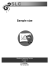### Small-Sample C.I.s for one- sample, two-sample and (matched) paired data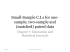### Email: [email protected]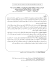### (7 pages) APRIL 2013 12. What is the difference between small sample and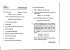### DETERMINING SAMPLE SIZE FOR RESEARCH ACTIVITIES ROBERT V. KREJCIE### measure attention